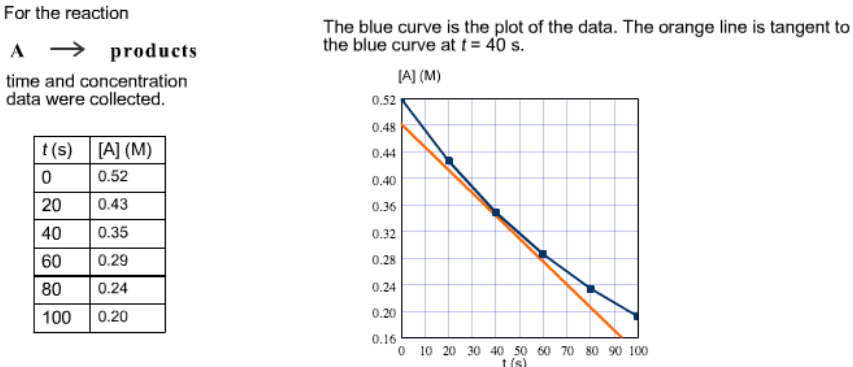# Problem: Approximate the instantaneous rate of this reaction at time  t = 40 s.

###### FREE Expert Solution
86% (483 ratings)
###### Problem Details

Approximate the instantaneous rate of this reaction at time  t = 40 s.Frequently Asked Questions

What scientific concept do you need to know in order to solve this problem?

Our tutors have indicated that to solve this problem you will need to apply the Instantaneous Rate of Change concept. If you need more Instantaneous Rate of Change practice, you can also practice Instantaneous Rate of Change practice problems.

What is the difficulty of this problem?

Our tutors rated the difficulty ofApproximate the instantaneous rate of this reaction at time ...as medium difficulty.

How long does this problem take to solve?

Our expert Chemistry tutor, Dasha took 3 minutes and 8 seconds to solve this problem. You can follow their steps in the video explanation above.

What professor is this problem relevant for?

Based on our data, we think this problem is relevant for Professor Schwab's class at APP STATE.